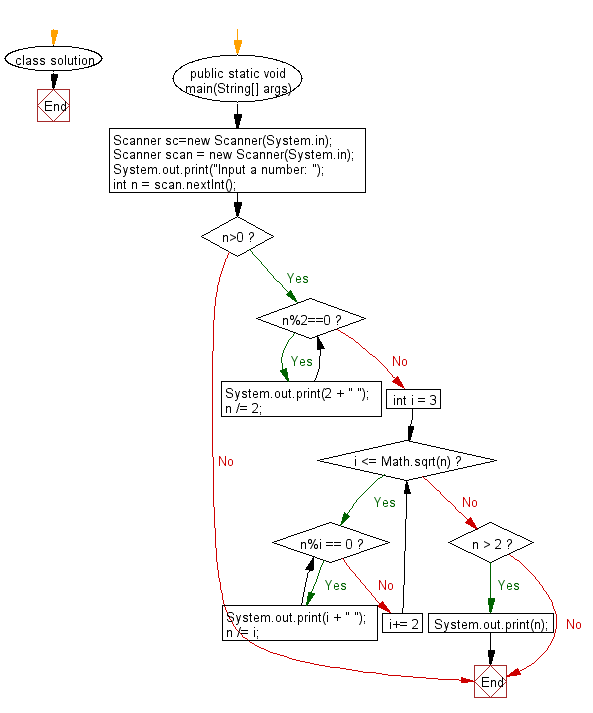﻿ Java Math Exercises: Print all prime factors of a given number - w3resource# Java Math Exercises: Print all prime factors of a given number

## Java Math Exercises: Exercise-26 with Solution

Write a Java program to print all prime factors of a given number.

Sample Solution:

Java Code:

``````import java.util.*;
class solution {
public static void main(String[] args)
{
Scanner sc=new Scanner(System.in);
Scanner scan = new Scanner(System.in);
System.out.print("Input a number: ");
int n = scan.nextInt();
if (n>0)
{
while (n%2==0)
{
System.out.print(2 + " ");
n /= 2;
}

for (int i = 3; i <= Math.sqrt(n); i+= 2)
{
while (n%i == 0)
{
System.out.print(i + " ");
n /= i;
}
}
if (n > 2)
System.out.print(n);
}
}
}
``````

Sample Output:

```Input a number:  78
2 3 13
```

Flowchart:Java Code Editor:

What is the difficulty level of this exercise?

﻿

## Java: Tips of the Day

Parsing dates:

```import java.io.*;
import java.util.*;
import java.text.*;

String s = "2001/09/23 14:39";

SimpleDateFormat formatter = new SimpleDateFormat ("yyyy/MM/dd H:mm");
Date d = formatter.parse(s, new ParsePosition(0));
```